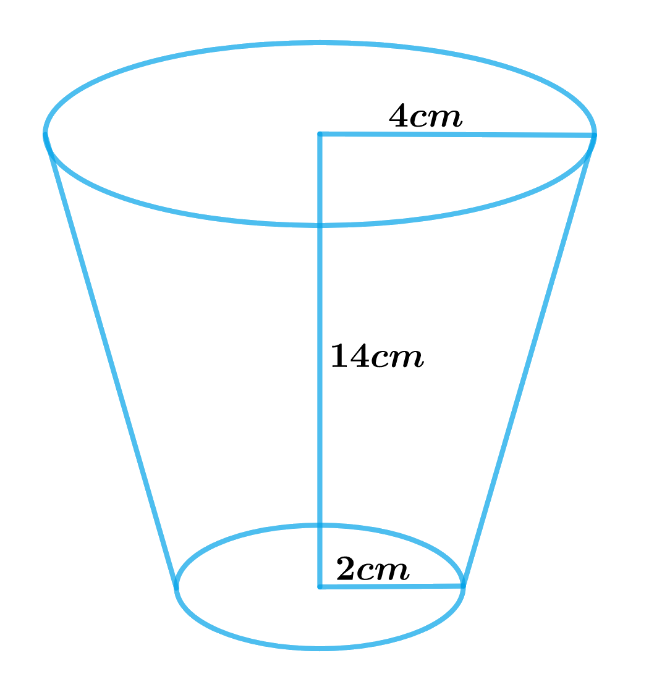# Ex.13.4 Q1 Surface Areas and Volumes Solution - NCERT Maths Class 10

Go back to  'Ex.13.4'

## Question

A drinking glass is in the shape of a frustum of a cone of height $$14\,\rm{cm.}$$ The diameters of its two circular ends are $$4\,\rm{cm}$$ and $$2\,\rm{cm.}$$ Find the capacity of the glass.

Video Solution
Surface Areas And Volumes
Ex 13.4 | Question 1

## Text Solution

What is Known?

A drinking glass is in the shape of a frustum of a cone of height is  $$14\, \rm{cm}.$$

The diameter of its two circular ends are $$4\, \rm{cm}$$ and $$2\,\rm{cm}.$$

What is Unknown?

The capacity of the glass

Reasoning:

Draw a figure to visualize the shape betterSince the glass is in the shape of a frustum of a cone

Therefore, the capacity of the glass $$=$$ Volume of frustum of a cone

We will find the capacity of the glass by using formulae

Volume of frustum of a cone\begin{align} = \frac{1}{3}\pi h\left( {r_1^2 + r_2^2 + {r_1}{r_2}} \right)\end{align}

where $$r1, r2$$ and $$h$$ are the radii and height of the frustum of the cone respectively.

Steps:

Height of glass,$$h = 14 \rm cm$$

Radius of the larger base,\begin{align} {r_1} = \frac{{4 \rm cm}}{2} = 2 \rm cm\end{align}

Radius of the smaller base,\begin{align} {r_2} = \frac{{2 \rm cm}}{2} = 1 \rm cm\end{align}

The capacity of the glass $$=$$ Volume of frustum of a cone

\begin{align}&= \frac{1}{3}\pi h\left( {r_1^2 + r_2^2 + {r_1}{r_2}} \right)\\&= \begin{bmatrix} \frac{1}{3} \times \frac{{22}}{7} \times 14 \rm cm \times \\ \begin{pmatrix} \left( 2 \rm cm \right)^2 + \left( 1 \rm cm \right)^2 \\ + 2 \rm cm \times 1cm \end{pmatrix} \end{bmatrix} \\&= \begin{bmatrix} \frac{{44}}{3} \rm cm \times\\ \begin{pmatrix} 4 \rm c{m^2} + 1c{m^2} \\ + 2 \rm c{m^2} \end{pmatrix} \end{bmatrix} \\&= \frac{{44}}{3} \rm cm \times 7 \rm c{m^2}\\&= \frac{{308}}{3} \rm c{m^2}\\&= 102\frac{2}{3} \rm c{m^2}\end{align}

Therefore, the capacity of the glass is \begin{align}102\frac{2}{3}\,\rm{cm^3}\end{align}

Learn from the best math teachers and top your exams

• Live one on one classroom and doubt clearing
• Practice worksheets in and after class for conceptual clarity
• Personalized curriculum to keep up with school